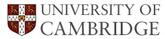# The OTIS KING Calculator

## H. K. D. H. Bhadeshia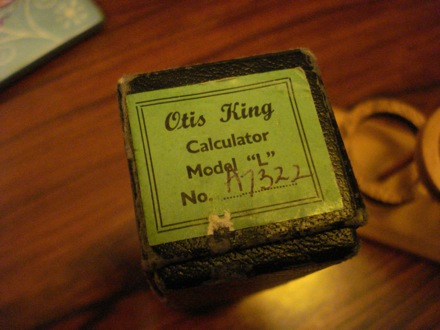Before electronic calculators, slide rules were used to do quick estimates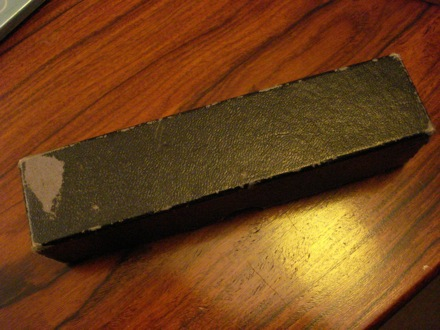The accuracy of a slide rule increases with its length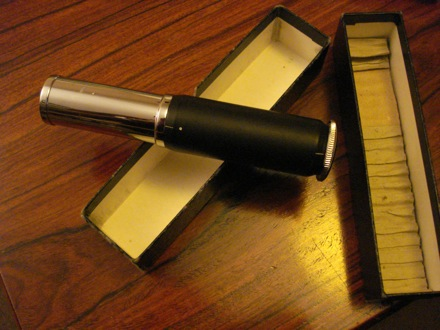This is an ingenious slide rule, short in absolute length but very accurate.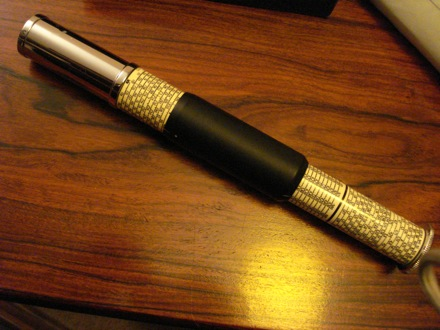This is because the slide rule is inscribed as a spiral around a cylinder.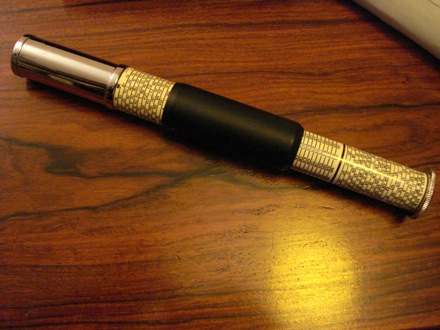The central cylinder slides along the axis.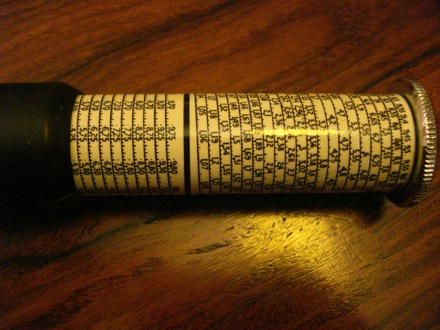Notice the sprial. This is the top half.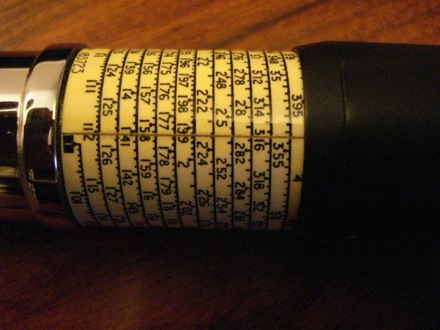The lower half.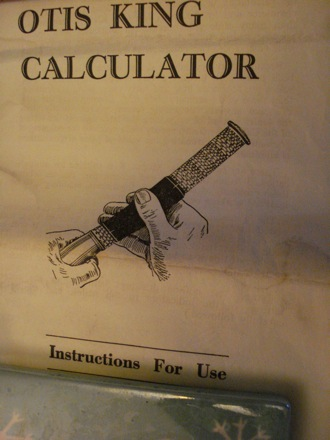The device was known as the OTIS KING calculator, accurate to at least three places after the decimal.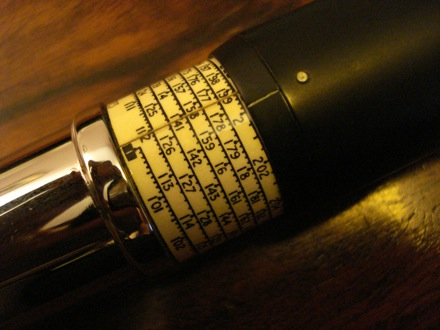To multiply 2 by 4, the lower cursor is positioned on the 2.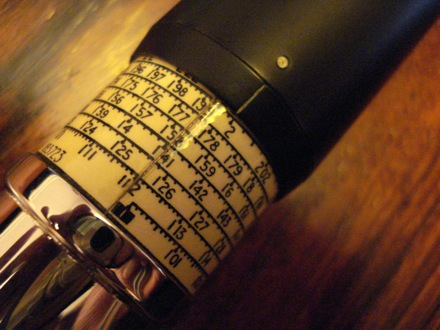The lower cursor is positioned on '2'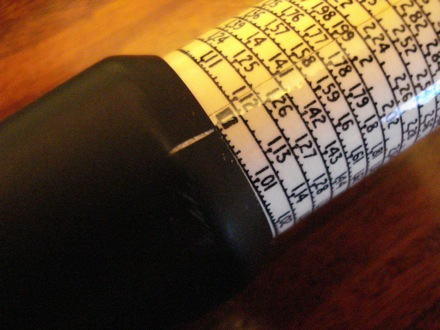The top spiral is then rotated so that the upper cursor matches '1'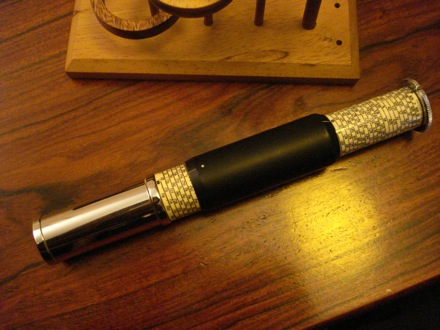This is the position of the cylinder at this stage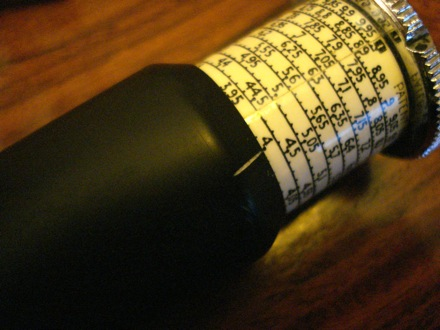The cylinder is then shifted upwards until the upper cursor is on '4'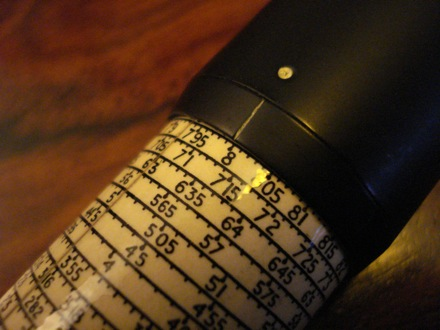The answer is revealed by the position of the lower cursor as '8'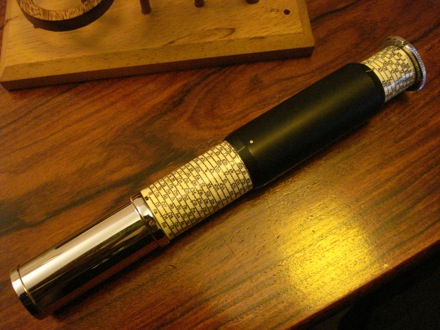This is the calculator with the correct positions for 2 x 4 =8